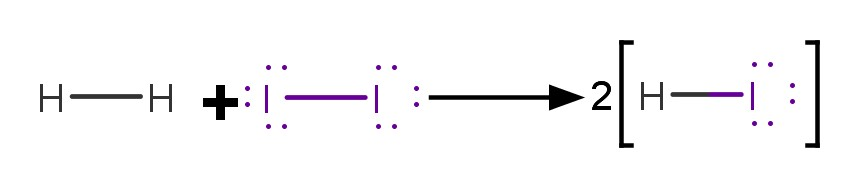# Problem: Gases react explosively if the heat released when the reaction begins is sufficient to cause more reaction, which leads to a rapid expansion of the gases. Use bond energies to calculate ΔH° of the following reaction, and predict which occurs explosively:(b) H2(g) + I2(g) ⟶2HI(g)

###### FREE Expert Solution

Bonds present from balanced equation:###### Problem Details

Gases react explosively if the heat released when the reaction begins is sufficient to cause more reaction, which leads to a rapid expansion of the gases. Use bond energies to calculate ΔH° of the following reaction, and predict which occurs explosively:

(b) H2(g) + I2(g) ⟶2HI(g)